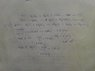# Reaction of KCI and MgCl2 with AgNO3

• cliffordlim
AgClSorry guys I'm stuck after calculating the moles AgCl , then I have no idea since both MgCl2 and KClIn summary, the mixture consisted of 1.2505 grams of KCI and MgCl2, and an excess of AgNO3 was added. The chloride ions were precipitated as AgCl. The mass of AgCl obtained was 2.5788 grams.f

#### cliffordlim

You have to show your attempts, this is a forum policy. Also, please don't ignore the template.
A mixture consisting of KCI and MgCl2 weights 1.2505 g. The mixture was
dissolved in water and an excess of AgNO3 was added. The chloride ions
were precipitated as AgCl. The mass of AgCl obtained was 2.5788 g.
Calculate the percentages of KCI and MgCl2 in the original mixture.

You are fairly new here. See the general site instructions – we do not help students who show no effort at all and also there is a template for homework problems.

Also it is not possible that you do not have a book that explains the general principles (atomic weights and molecular formulae etc.) as well as worked examples of how you would calculate the amount of Cl that was in so many grams of AgCl, which is the first part of the problem.

Can you show us what you already have done towards the solution of this problem?

#### Attachments

•13292854_474251089440635_868770269_n.jpg
47.8 KB · Views: 2,080
Sorry guys I'm stuck after calculating the moles AgCl , then I have no idea since both MgCl2 and KCl

Sorry guys I'm stuck after calculating the moles AgCl , then I have no idea since both MgCl2 and KCl
Sounds like you were cut off in mid-sentence. Did you want to finish writing down your thoughts?

First of all your 3rd chemical reaction is not correct, you cannot always add chemical reactions the same way you add equations in math.

Suppose x are the moles of KCI and y are the moles of MgCl2 present in the initial mixture that weights 1.2505g. What equation can you make involving x, y, the molecular weights and the total mass of 1.2505g?

Sounds like you were cut off in mid-sentence. Did you want to finish writing down your thoughts?
as refer the photo https://www.physicsforums.com/attachments/13292854_474251089440635_868770269_n-jpg.101434/
AgNO3 + KCl ---> AgCl + KNO3 ...(i)
2AgNO3 + MgCl2 ----> 2AgCl + Mg(NO3)2 ...(ii)
mole of AgCl 2.5788g/143.28 = 0.018mol , Assume
since I 1 mol from Chloride from equation (i) and 2 mole from equation (ii) total 3 mole
mass of KCl 0.018/3 x 74.55g mol-1 = 0.477g
mass of MgCl2 0.018/3 x 95.2g mol-1 = 0.5712g
after calculating the mass 0.477g KCl + 0.5712g = 1.0482g but this amount is not equal to orginal mass .1.2505g

First of all your 3rd chemical reaction is not correct, you cannot always add chemical reactions the same way you add equations in math.

Suppose x are the moles of KCI and y are the moles of MgCl2 present in the initial mixture that weights 1.2505g. What equation can you make involving x, y, the molecular weights and the total mass of 1.2505g?
Thanks you , next time I will show the solution / effort . I'm fairly new here ...

as refer the photo https://www.physicsforums.com/attachments/13292854_474251089440635_868770269_n-jpg.101434/
AgNO3 + KCl ---> AgCl + KNO3 ...(i)
2AgNO3 + MgCl2 ----> 2AgCl + Mg(NO3)2 ...(ii)
mole of AgCl 2.5788g/143.28 = 0.018mol , Assume
since I 1 mol from Chloride from equation (i) and 2 mole from equation (ii) total 3 mole
mass of KCl 0.018/3 x 74.55g mol-1 = 0.477g
mass of MgCl2 0.018/3 x 95.2g mol-1 = 0.5712g
after calculating the mass 0.477g KCl + 0.5712g = 1.0482g but this amount is not equal to orginal mass .1.2505g

The correct way to think is that from 1 mole of KCl you get 1 mole of AgCl cause that's what (i) tell us among other things, hence from x moles KCl you ll get x moles of AgCl. But you ll get additional moles of AgCl from (ii). Can you express those additional moles in terms of y? The total moles of AgCl will be equal to 0.018

The correct way to think is that from 1 mole of KCl you get 1 mole of AgCl cause that's what (i) tell us among other things, hence from x moles KCl you ll get x moles of AgCl. But you ll get additional moles of AgCl from (ii). Can you express those additional moles in terms of y? The total moles of AgCl will be equal to 0.018
ermm..it is
1 mole of KCl = 1 mole of AgCl
(0.018-x) mole of KCl = 0.018 mole of AgCl

1 mole of MgCl2 = 2 mole of AgCl
1/2 (0.018-y) mole of MgCl = 0.018 mole of AgCl
??
or
1 mole of KCl = 1 mole of AgCl
x mole of KCl = x mole of AgCl

1 mole of MgCl2 = 2 mole of AgCl
1/2y-x mole of MgCl2 =

ermm..it is
1 mole of KCl = 1 mole of AgCl
(0.018-x) mole of KCl = 0.018 mole of AgCl

1 mole of MgCl2 = 2 mole of AgCl
1/2 (0.018-y) mole of MgCl = 0.018 mole of AgCl
??
or
1 mole of KCl = 1 mole of AgCl
x mole of KCl = x mole of AgCl

1 mole of MgCl2 = 2 mole of AgCl
1/2y-x mole of MgCl2 =
Sorry can't follow what you doing here...i ve posted two questions one in post #9 and one in post #12, if you can successfully answer those questions you ll make two linear equations each one involving x (the moles of KCl) and y(the moles of MgCl2) . Then you can easily solve the system of those two equations to obtain x and y.

•cliffordlim